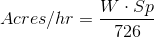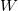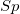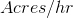You have no items in your shopping cart.
Search

# Travelling Irrigator Irrigation Area Rate

This calculator finds the number of irrigated acres per hour, based on lane spacing, and travel speed. Lane spacing is the distance between the pulls on a traveling sprinkler. The travel speed is the rate that the traveling sprinkler is reeled in.

## Traveling Sprinkler Irrigation Area Rate

Lane Spacing:
Travel Speed:
Total Irrigated Acres per Hour:
acres/hr

### The Equation

This calculator uses this formula to determine the area irrigated per hour by a traveling sprinkler.When:= The width between pulls of the traveling sprinkler (ft)= The speed that the traveling sprinkler is reeled in (ft/min)= Total irrigated area per hour (acres/hr)

Reference: Washington State University